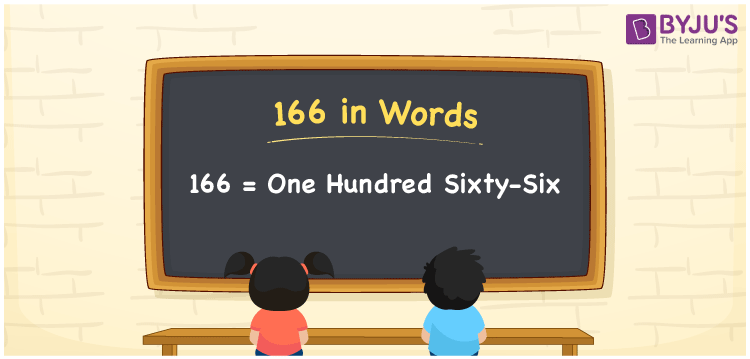# 166 in Words

166 in words is one hundred sixty-six. The numeral 166 is a three-digit number and it appears after 165 and before 167. The transformation of numbers into words is very simple if you know the place values of the number. Also, the number 166 is a cardinal number. For example, the cost of a pen is Rs. 166.

 166 in Words: One Hundred Sixty-six. One Hundred Sixty-six in Numerical Form: 166.

## 166 in English Words## How to Write 166 in Words?

Go through the below place value table for the numeral 166.

 Hundreds Tens Ones 1 6 6

The expanded form of 166 is as follows:

= 1 × Hundred + 6 × Ten + 6 × One

= 1 × 100 + 6 × 10 + 6 × 1

= 100 + 60 + 6

= 166

= One hundred sixty-six

Hence, 166 in words is one hundred sixty-six.

166 in words – One hundred sixty-six

Is 166 an odd number? – No

Is 166 an even number? – Yes

Is 166 a perfect square number? – No

Is 166 a perfect cube number? – No

Is 166 a prime number? – No

Is 166 a composite number? – Yes

## Frequently Asked Questions on 166 in Words

Q1

### Write 166 in English words.

166 in words is one hundred sixty-six.

Q2

### Simplify 160 + 6, and express it in words.

Simplifying 160 + 6, we get 166. Hence, 166 in words is one hundred sixty-six.

Q3

### Is 166 a prime number?

No, 166 is not a prime number.